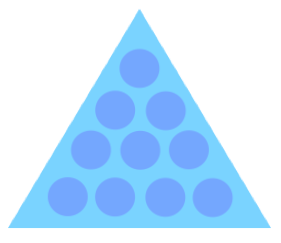Is 121 a triangular number?Here we will define and determine if 121 is a triangular number, also known as a triangle number. First, note that an equilateral triangle is a triangle where the length of the three sides are the same, and the three internal angles of the triangle are 60 degrees. An equilateral triangle can look like this:Take notice of the symmetry of the dots inside the triangle above. Each side of the triangle has the same number of dots, and one dot is added to each row of dots.

121 is a triangular number if we can arrange all 121 dots or objects in perfect symmetry to make an equilateral triangle. To determine if a number (n) is a triangular number, the following equation must be true:

(8 × n) +1 = Integer

When we enter 121 for n in our formula, we get the following result:

(8 × 121) +1 ≈ 31.1288

31.1288 is not an integer (whole number), therefore the answer to "Is 121 a triangular number?" is as follows:

121 = Not a triangular number

Since 121 is not a triangular number, you cannot use 121 objects or dots to create a symetric equilateral triangle similar to the one pictured above on this page.

Triangular Number Calculator
Check if another number is a triangular number.

Is 122 a triangular number?
Go here to find out if the next number on our list is a triangular number.